Open in App
Not now

# Small World Model – Using Python Networkx

• Last Updated : 05 Aug, 2022

In this article, we will learn how to create a Small World Network using Networx module in Python. Before proceeding that let’s first understand some basics about Small World Phenomenon. What is Small World Phenomenon ? Small World Phenomenon is the study and notion that we are all connected via a small number of edges. There have been three notable experiments to prove the Small World Phenomenon :

1. Milgram Small World Phenomenon Experiment : 296 randomly chosen persons were asked to forward a letter to a ‘target’ person (a Stockbroker in Boston). The letter was to be sent directly to him if the person was known personally, otherwise, the letter was to be sent to someone who has a higher probability of knowing him personally. 64 letters reached the target person with a median length of 6 ie. on average a random person was connected to the target person via 6 people in between.
2. Microsoft Instant Messenger Experiment : There are 240 million active users of Microsoft Instant Messenger. They are connected if two users were engaged in a 2-way communication over the period of a month. For any two random people, the median distance was 7 i.e. 2 random people were connected via 7 intermediate connections.
3. Facebook based Experiment : The experiment conducted by Facebook calculated the average path length to be 5.28 in 2008 whereas it reduced to be 4.74 in 2011.

In 1998, Duncan Watts and Steven Strogatz furthered the study of Small World Model by publishing a research paper titled “Collective Dynamics of Small World Networks”. They studied three different kinds of networks:

1. A network of film actors, where the individual nodes were film actors and they were connected only if the actors appeared in the same movie.
2. A power grid network where nodes are generators, transformers and substations and two nodes are linked if they have a transmission line between them.
3. A network where nodes are neurons and two nodes are linked if they have a synapse or a gap junction.

They concluded that Small World Networks generally has low average path length but high clustering coefficient.

## How can Small World Networks be formed ?

Watts and Strogatz came up with a model about how to construct Small World Networks. Let there be n nodes, where each node is connected to m nearest neighbors, this is known as Regular Lattice and looks like as shown in the figure below, where n = 10 and m = 4.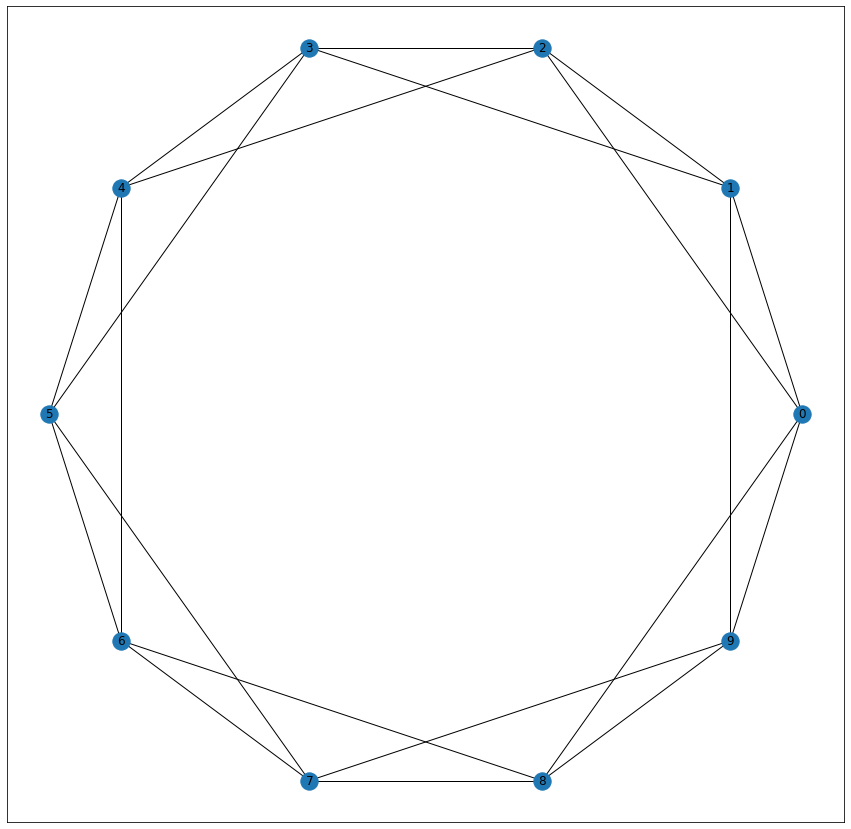Consider each edge (u, v) and with probability p, select a node w at random and rewire the edge (u, v) so that it becomes (u, w). For p = 0, the Regular Lattice retains its structure and has a high average distance and high clustering. For p = 1, a Random Network is formed with small average distance and low clustering. It looks like the figure shown below, where n = 10, m = 4 and p = 1.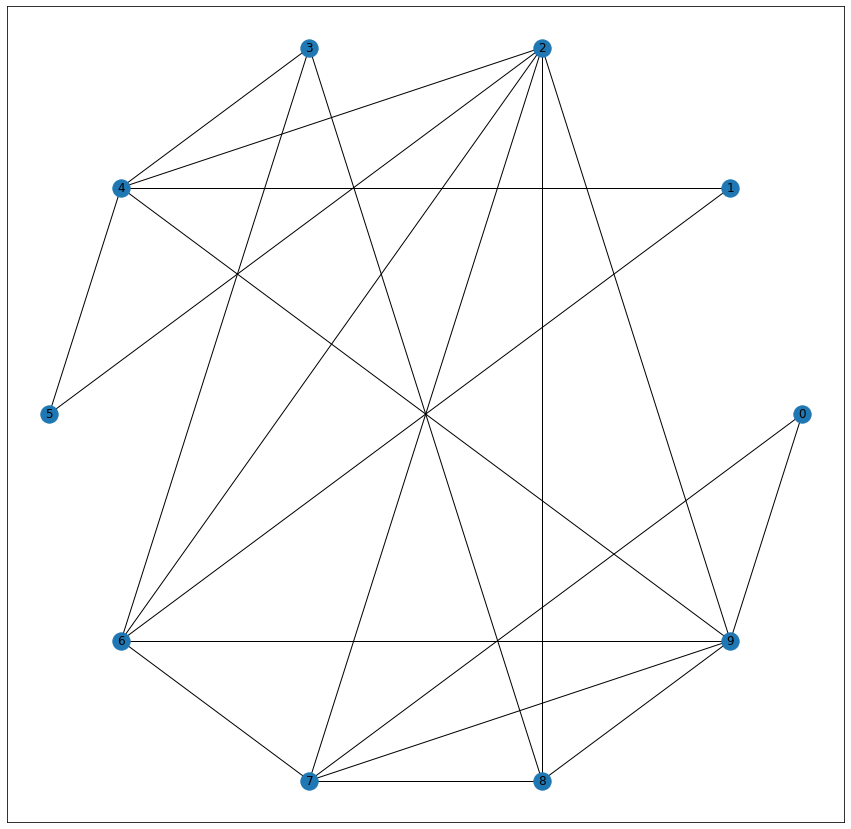For an intermediate value of p, we would get an ideal Small World Network with small average distance and high clustering. For Python, we can easily construct a Small World Network using Networkx.

## Python3

 `import` `networkx as nx``import` `matplotlib.pyplot as plt` `G ``=` `nx.watts_strogatz_graph(n ``=` `10``, m ``=` `4``, p ``=` `0.5``)``pos ``=` `nx.circular_layout()` `plt.figure(figsize ``=` `(``12``, ``12``))``nx.draw_networkx(G, pos)`

Output: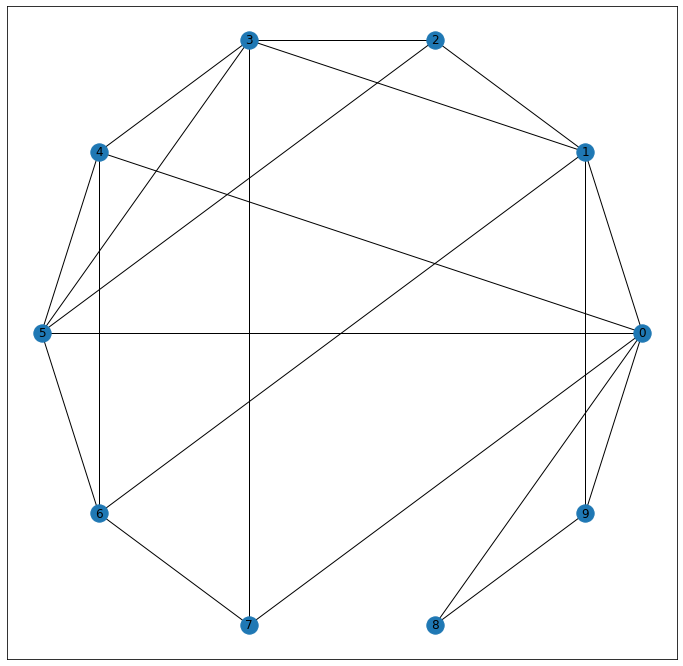The resultant Small World Network maybe a disconnected Graph. If we wish to get a connected Graph, we can modify line number 4 of the above code as follows:

## Python3

 `G ``=` `nx.connected_watts_strogatz_graph(n``=``10``, m``=``4``, p``=``0.5``, t``=``20``)`

It runs the original function t times (in this case t = 20) till a connected network is achieved. It will give the following network: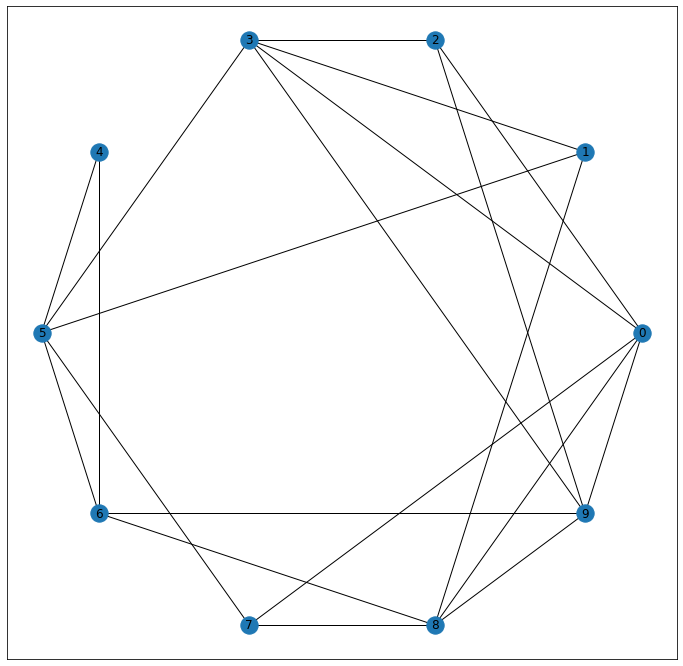## Python3

 `G ``=` `nx.newman_watts_strogatz_graph(n``=``10``, m``=``4``, p``=``0.5``)`

The above code will run a similar model but add new edges with probability p instead of rewiring already existing edges. It will produce the following network: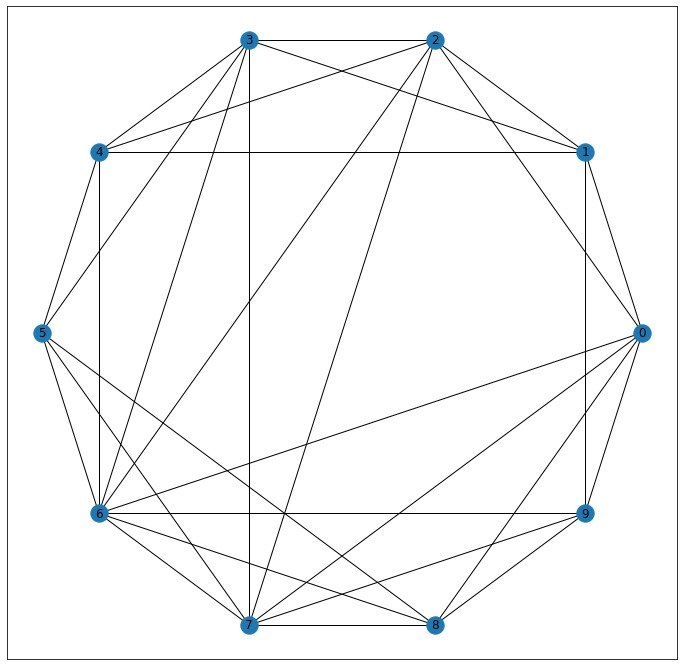My Personal Notes arrow_drop_up